# Flag space

(diff) ← Older revision | Latest revision (diff) | Newer revision → (diff)

A projective-space whose metric is defined by an absolute consisting of a collection of-planes,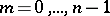, imbedded in one another, called a flag; a flag space is denoted by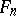. The absolute of a flag space can be obtained from the absolutes of Galilean or pseudo-Galilean spaces by means of a passing to the limit in the quadrics of the absolutes. In particular, the flag (absolute) of the spaceconsists of a-plane, in which lies a line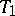(a Euclidean line), and on the line a point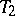. The plane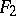is a projective-plane with a distinguished line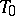and a distinguished pointand coincides with Yaglom's Galilean plane.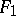is a projective line with a distinguished point, and is the same as the Euclidean line.

If one chooses an affine coordinate system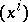in a flag spaceso that the vectors of the lines passing through the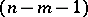-plane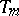are defined by the condition, then one takes the numberas the distance between the points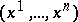and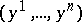; if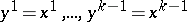, then the distance is defined by the number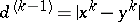.

Lines that intersect the-plane and not the-plane are called lines of order.

Motions of a flag space are collineations mapping the absolute into itself. The motions of a flag space form a subgroup of the affine transformations of an affine-space, and this group of motions of a flag space is a Lie group.

A spaceis self-dual. As the value of the angle between two-planes one takes the distance between the points dual to these planes.

A flag space is a special case of a semi-elliptic space. In particular, the flag spaceis the same as the-space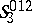. The flag-space is the unique space with parabolic distance metrics on lines, in semi-planes and in bundles of planes.

How to Cite This Entry:
Flag space. Encyclopedia of Mathematics. URL: http://encyclopediaofmath.org/index.php?title=Flag_space&oldid=14163
This article was adapted from an original article by L.A. Sidorov (originator), which appeared in Encyclopedia of Mathematics - ISBN 1402006098. See original article